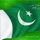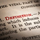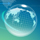# remainder Balochi Meaning#### Brahui Dictionary

English to Brahui Dictionary

remainder

بخال#### Definitions

English definition for remainder

1. n. a piece of cloth that is left over after the rest has been used or sold

2. n. the number that remains after subtraction; the number that when added to the subtrahend gives the minuend

3. n. the part of the dividend that is left over when the dividend is not evenly divisible by the divisor

4. n. something left after other parts have been taken away

5. v. sell cheaply as remainders

In mathematics, the remainder is the amount "left over" after performing some computation. In arithmetic, the remainder is the integer "left over" after dividing one integer by another to produce an integer quotient (integer division).